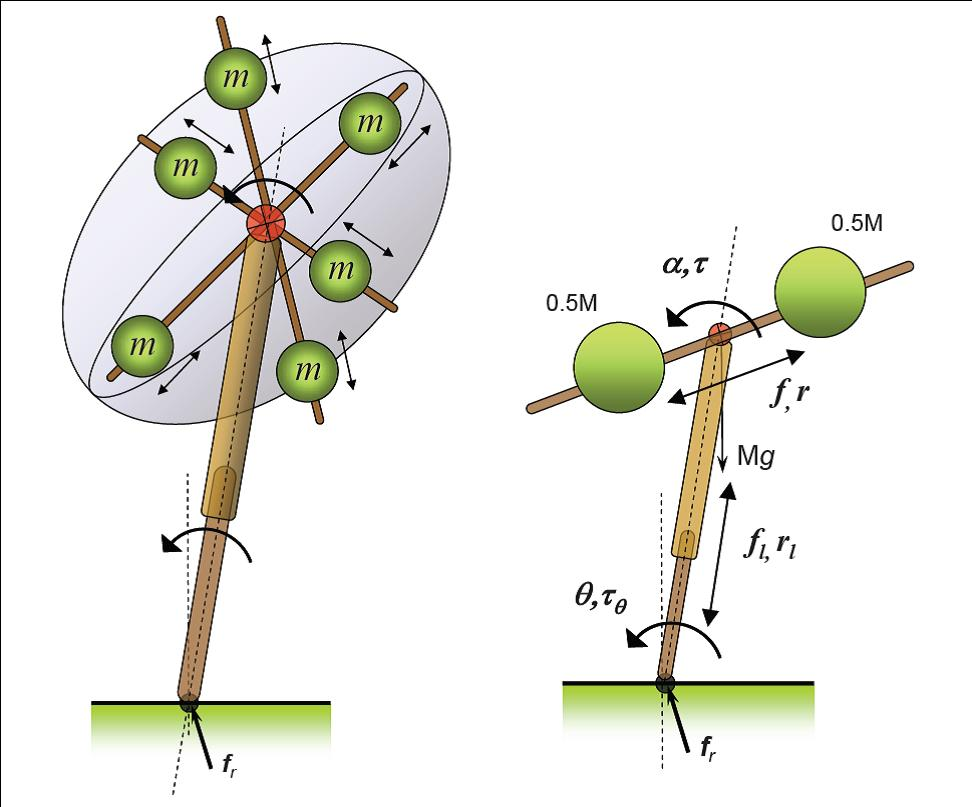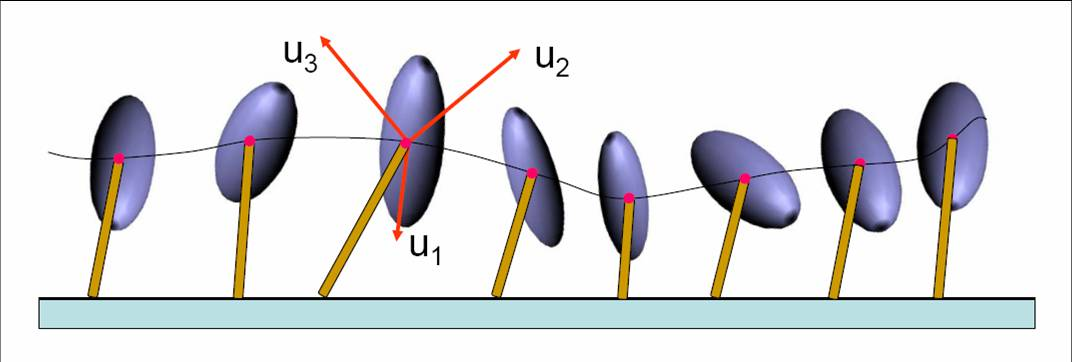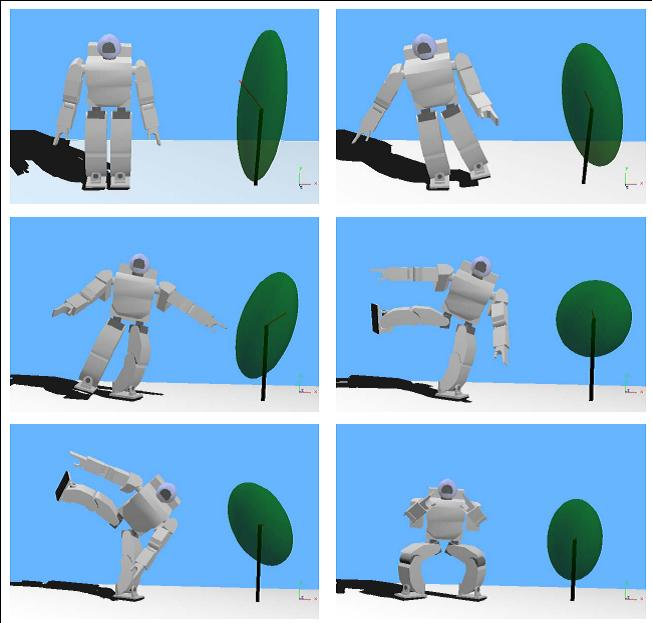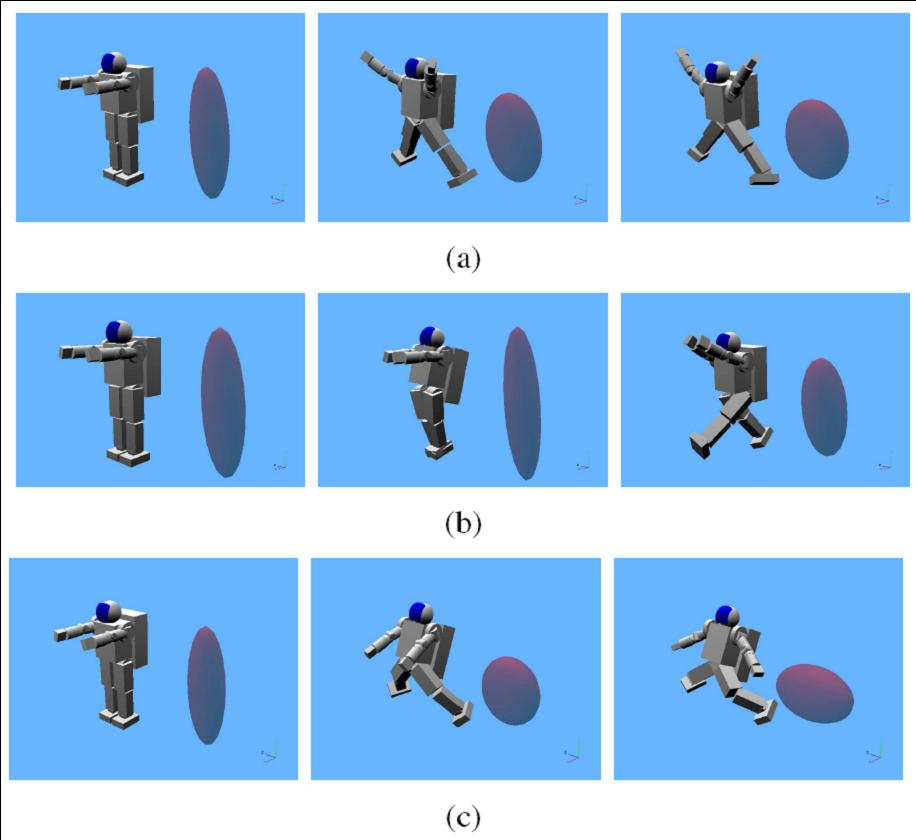Simple model for analysis and control of humanoid robots
ambarish.com Ambarish Goswami's Current Research
 Home/ Current Research/ Simple humanoid models for balance and control

## Reaction Mass Pendulum (RMP):Simple humanoid model for balance and control

 Reduced biped models such as the different variations of the inverted pendulum models have been very beneficial for the analysis and understanding of human and humanoid gait. These models allow us to ignore the movements of the individual limbs of the humanoid and instead focus on two important points - the center of pressure (CoP) and the center of mass (CoM) - and the line joining them. By focusing attention to the fundamental aspects of humanoid dynamics, such models open the way to new classes of control laws, which would otherwise be difficult or impossible to conceive. Once the basic characteristics of the control strategy is formulated in the reduced-dimensional space, where intuition is strong, one needs to "map" the strategy back to the full dynamic model of the humanoid for an implementation ready control law. While useful in their own right, a limitation of the above models is that they represent the entire humanoid body only as a point mass and do not characterize the significant centroidal moment of inertia of the humanoid body. The centroidal moment of inertia is a property of the distributed masses of the robot limbs (head, arms, legs, etc) away from the CoM. We have demonstrated that a humanoid's state of balance is closely related to its rotational equilibrium which, in turn, is dependent on its angular momentum rate change. The centroidal moment of inertia directly contributes to the centroidal angular momentum and its rate of change. Direct manipulation of momenta is becoming a reasonable, and sometimes preferable, way to control a robot. We present the Reaction Mass Pendulum (RMP) model as an extension of the traditional inverted pendulum models. The RMP model explicitly models the robot's extended intertia by means of its instantaneous centroidal composite rigid body inertia (CCRBI) matrix. CCRBI matrix is identical to the so-called locked inertia and represents the aggregate rigid body inertia of entire humanoid computed at its CoM, assuming that its joints are temporarily locked. It is a single rigid-body approximation of the multi-body humanoid. An RMP consists of two components, a ``leg'' that joins the CoP and the CoM, and an ellipsoidal ``body'' -- the abstracted reaction mass -- that characterizes the generalized inertia of the entire robot projected at the CoM. As the robot moves in space, so does the RMP, resulting in a continuous movement of the CoP and CoM. All limb movements of the robot affect its centroidal moment of inertia, which is captured by the changing shape, size and orientation of the ellipsoidal reaction mass. The reaction wheel or inertia wheel is one of a number of standard momentum exchange devices that are used to control satellite orientation. An actuated reaction wheel attached to a rigid rod becomes a reaction wheel pendulum.Conceptual mechanical realization of the 3D reaction mass pendulum (RMP) and its planar version, the 2D reaction wheel pendulum. The ellipsoid of the 3D RMP can be reduced to three pairs of equal point masses at different radial distances that are actuated to slide on their linear tracks. The overall frame consisting of the three pairs of linear tracks form the skeleton which can be actuated in three rotational degrees of freedom (dof). For the 2D model the distance between the two point masses is 2r.The 3D reaction mass has continuously variable inertia. At any given configuration of the robot, the centroidal rigid body inertia can be reduced to an ellipsoid.Snapshots of HOAP2 robot performing Sumo-style motion superposed with corresponding RMP models. The reaction mass geometry undergoes significant changes during this motion Inertia Shaping An interesting application of the RMP modeling approach is what we call inertia shaping of an articulated chain. Inertia shaping is a high-level approach to precisely control the aggregate kinodynamic characteristics of an articulated chain by controlling its CRB inertia. Given a desired CRB inertia from the RMP model, the humanoid should make a proper pose to achieve this goal. This can be posed as an inverse kinematics problem with the desired CRB inertia constraints. Since we have derived the CRB inertia Jacobian, the inverse kinematics problem can be solved by any suitable optimization algorithm. The simplest solution will be updating desired joint angles using the pseudo-inverse of CRB inertia Jacobian. The following figure presents three examples of inertia shaping on a non-contacting biped floating in space (say, a humanoid astronaut). The robot is given three different commands, shown in series a, b, and c, respectively, to try to match its own CRB inertia to a desired CRB inertia. Starting from an initial configuration, the robot moves its joints such that the cost function, the Frobenius norm of the difference between the two inertias, is minimized.Demonstration of the inertia shaping technique on a non-contacting biped robot floating in space. A floating robot has no CoP, so the RMP reduces to simply the ellipsoidal reaction mass In Fig. a, the desired inertia components along all three axes are equal and large. Hence the robot tries to "expand" in all directions. In Fig. b, the desired inertia along Y-axis (vertical) is big, and the other directions are very small. In Fig. c, robot tries to make its inertia along X and Z larger at the cost of Y-direction. This simulation demonstrates the important point of effectively controlling a complex biped with a very simple control law. While the robot model has 27 dofs, the control law deals with only three variables which are the three diagonal elements of the robot's rotational inertia.

A list of my papers on this topic:

• A. Sanyal and A. Goswami,
Dynamics and Control of the Reaction Mass Pendulum (RMP) as a 3D Multibody System: Application to Humanoid Modeling,
2011 ASME Dynamic Systems and Control Conference (DSCC), Arlington, VA, October 2011.

(pdf).

Abstract: Humans and humanoid robots are often modeled with different types of inverted pendulum models in order to simplify the dynamic analysis of gait, balance and fall. We have earlier introduced the Reaction Mass Pendulum (RMP), an extension of the traditional inverted pendulum models, which explicitly captures the variable rotational inertia and angular momentum of the human or humanoid. In this paper we present a thorough analysis of the RMP, which is treated as a 3D multibody system in its own right. We derive the complete kinematics and dynamics equations of the RMP system and obtain its equilibrium conditions. Next we present a nonlinear control scheme that stabilizes this underactuated system about an unstable set with a vertically upright configuration for the ``leg" of the RMP. Finally we demonstrate the effectiveness of this controller in simulation.

• A. Dutta and A. Goswami
Human postural model that captures rotational inertia,
The 33rd Annual Meeting of the American Society of Biomechanics ASB 2010, Providence, Rhode Island, USA, August, 2010.

(pdf).

Abstract: Inverted pendulum models have been very beneficial for the modeling and analysis of human gait and balance. These reduced models allow us to ignore the movements of the individual limbs, and instead, focus on two important points - the center of pressure (CoP) and the center of mass (CoM) - and the lean line that connects the two points. A limitation of the existing reduced models is that they represent the entire human body only as a point mass and do not characterize its moment of inertia. The rotational inertia is a property of the distributed masses of the limbs, and by ignoring it, un-natural constraints, such as zero angular momentum at the CoM and resultant ground reaction force (GRF) collinear with the lean line, are forced on to the model.

The Reaction Mass Pendulum (RMP) model extends the existing models by replacing the point mass with an extended rigid body - the abstracted 3D reaction mass - that characterizes the aggregate rotational inertia of the subject projected at the CoM. As the person moves through different limb configurations, the centroidal moment of inertia continuously changes, which is captured by the changing shape, size and orientation of the ellipsoidal reaction mass. We postulate that analysis of the rotational inertia especially in cases of pathological gait can provide additional insight. This is demonstrated with normative gait data from four able-bodied subjects and pathological gait data from one spinal cord injured subject.

• A. Goswami,
Kinematic and dynamic analogies between planar biped robots and the reaction mass pendulum (RMP) model,
Humanoids 2008, Daejeon, Korea, December 2008.

(pdf).

Abstract: In order to simplify dynamic analysis, humanoid robots are often abstracted with various versions of the inverted pendulum model. However, most of these models do not explicitly characterize the robot's rotational inertia, a critical component of its dynamics, and especially of its balance. To remedy this, we have earlier introduced the "Reaction Mass Pendulum (RMP)", an extension of the inverted pendulum, which models the rotational inertia and angular momentum of a robot through its centroidal composite rigid body (CCRB) inertia. However, we presented only the kinematic mapping between a robot and its corresponding RMP.

Focussing in-depth on planar mechanisms, here we derive the dynamic equations of the RMP and explicitly compute the parameters that it must possess in order to establish equivalence with planar compass gait robot. In particular, we show that, a) an angular momentum equality between the robot and RMP does not necessarily guarantee kinetic energy equality, and b) a cyclic robot gait may not result in a cyclic RMP movement.

The work raises the broader question of how quantitatively similar the simpler models of humanoid robot must be in order for them to be of practical use.
Humanoids2009 poster

• S-H. Lee and A. Goswami,
Reaction Mass Pendulum (RMP): An explicit model for centroidal angular momentum of humanoid robots,
IEEE Int. Conf. on Robotics and Automation, Rome, Italy, April 2007.

(pdf).

Abstract: A number of conceptually simple but behaviorrich “inverted pendulum” humanoid models have greatly enhanced the understanding and analytical insight of humanoid dynamics. However, these models do not incorporate the robot’s angular momentum properties, a critical component of its dynamics. We introduce the Reaction Mass Pendulum (RMP) model, a 3D generalization of the better-known reaction wheel pendulum. The RMP model augments the existing models by compactly capturing the robot’s centroidal momenta through its composite rigid body (CRB) inertia. This model provides additional analytical insights into legged robot dynamics, especially for motions involving dominant rotation, and leads to a simpler class of control laws. In this paper we show how a humanoid robot of general geometry and dynamics can be mapped into its equivalent RMP model. A movement is subsequently mapped to the time evolution of the RMP. We also show how an “inertia shaping” control law can be designed based on the RMP.

Kangkang Yin contributed an important correction to the above paper. (Correction)
HOAP2 Sumo motion with simultaneous RMP
Fujitsu HOAP2 gait with simultaneous RMP

• M. Abdallah and A. Goswami,
A biomechanically motivated two-phase strategy for biped upright balance control,
IEEE Int. Conf. on Robotics and Automation, Barcelona, Spain, April 2005.

(pdf).

Abstract: Balance maintenance and upright posture recovery under unexpected environmental forces are key requirements for safe and successful co-existence of humanoid robots in normal human environments. In this paper we present a two-phase control strategy for robust balance maintenance under a force disturbance. The first phase, called the reflex phase, is designed to withstand the immediate effect of the force. The second phase is the recovery phase where the system is steered back to a statically stable “home” posture. The reflex control law employs angular momentum and is characterized by its counter-intuitive quality of “yielding” to the disturbance. The recovery control employs a general scheme of seeking to maximize the potential energy and is robust to local ground surface feature. Biomechanics literature indicates a similar strategy in play during human balance maintenance.

Recovery under potential energy control
Reflex and recovery against a 300N horizontal force
Robot balancing on a swaying table

• A. Goswami and V. Kallem,
Rate of change of angular momentum and balance maintenance of biped robots,
IEEE Int. Conf. on Robotics and Automation, New Orleans, April 2004.

(pdf).

Abstract: In order to engage in useful activities upright legged creatures must be able to maintain balance. Despite recent advances, the understanding, prediction and control of biped balance in realistic dynamical situations remain an unsolved problem and the subject of much research in robotics and biomechanics. Here we study the fundamental mechanics of rotational stability of multi-body systems with the goal to identify a general stability criterion. Our research focuses on H_G, the rate of change of centroidal angular momentum of a robot, as the physical quantity containing its stability information. We propose three control strategies using H_G that can be used for stability recapture of biped robots. For free walk on horizontal ground, a derived criterion refers to a point on the foot/ground surface of a robot where the total ground reaction force would have to act such that H_G = 0. This new criterion generalizes earlier concepts such as GCoM, CoP, ZMP, and FRI point, and extends their applicability.

• ##### Back to main page

Page last updated September 24, 2012